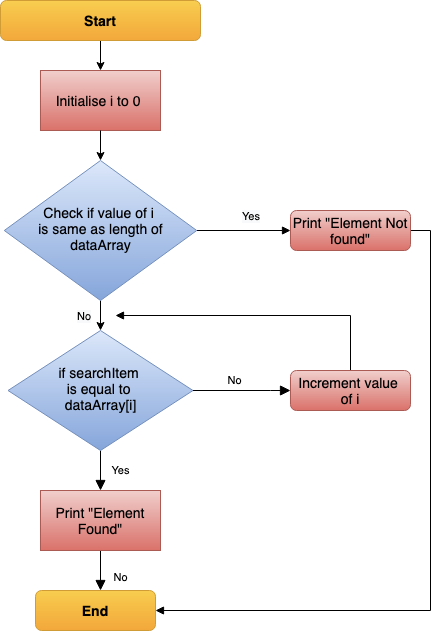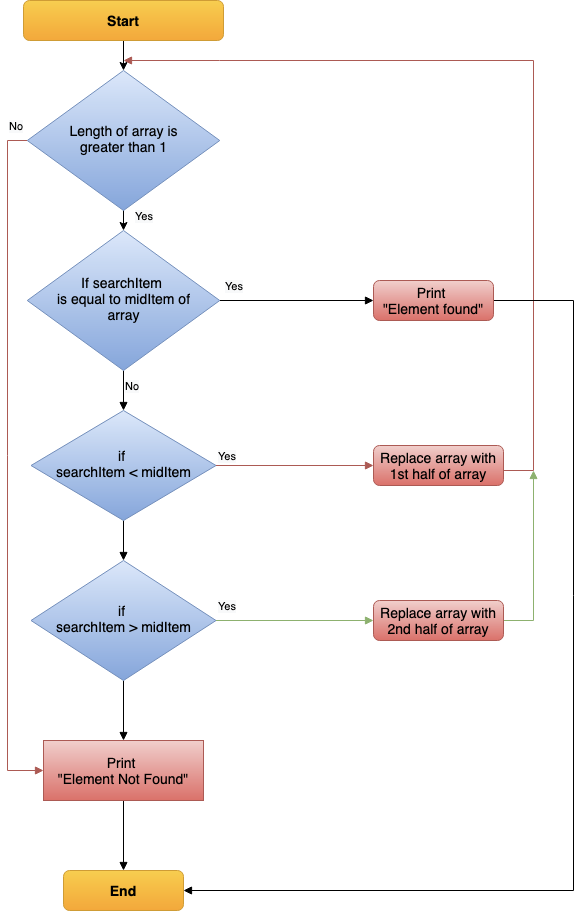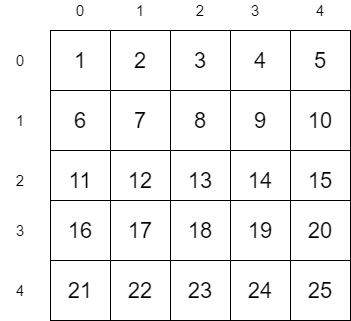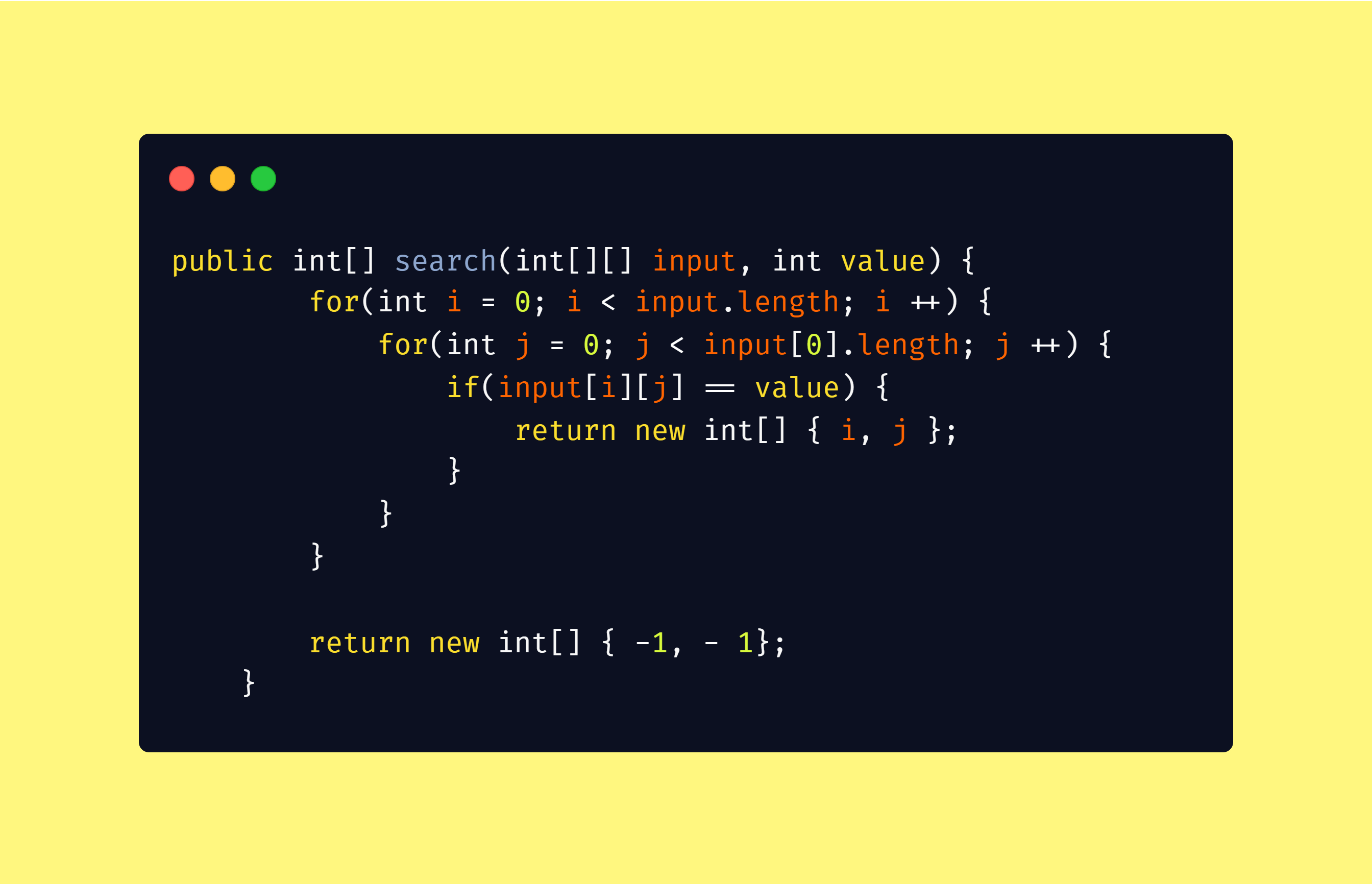1603767600

# Search Algorithms

Today, let us touch base on some fundamental concepts like search algorithms.

In simple terms, **searching **is a process of looking up a particular data record in the database or in the collection of items. A search typically answers as true or false whether the particular data in which we are referring is found or not and perform the next action accordingly.

Commonly used algorithms for search are:

• Linear search
• Binary search
• Interpolation search

Let us understand them in detail with some example

## Linear Search Algorithm

Linear Search Algorithm is the simplest and easiest form of the search algorithm. In this algorithm, a sequential search is made over all the items one by one to search for the targeted item. Each item is checked in sequence until the match is found. If the match is found, the searched item is returned otherwise the search continues till the end.

To make it easy to understand, let us see understand linear search using a flow diagramLinear Search — Data Flow

### Points to note:

• Does not need sorted array list
• Performs equality comparisons
• The time complexity is O(n)
• Time taken to search elements keeps increasing as the number of elements is increased.

## Binary Search Algorithm

In _Binary search algorithm, _begins with an interval covering the whole array and diving it in half. If the value of the search key is less than the item in the middle of the interval, narrow the interval to the lower half. Otherwise narrow it to the upper half. Repeatedly check until the value is found or the interval is empty.

To make it easy to understand, let us see understand binary search using flow diagram and example as below.Binary Search — Data Flow

### Points to note:

• The array needs to be sorted
• Performs ordering comparisons
• Time complexity to O(log n).
• Search is done to either half of the given list, thus cut down your search to half time

#sorting-algorithms #algorithms #data-structures #search-and-sort #searching-algorithm

## Buddha Community1609266954

If you’ve been running a business and working with a website for some time, you’ve probably experienced it firsthand. In order for you to have sales and for customers to be happy, you need to attract people to the site. You may know that traffic is important, but you may not know how to get it. Many people believe that a large amount of traffic can be easily attracted by SEO professional… if you pay well for it. But the truth is this: it’s easy enough to get traffic to your site for free, even if you’re on a budget! But if you have a small or medium-sized business, then, most likely, you do not have extra money.1603767600

## Search Algorithms

Today, let us touch base on some fundamental concepts like search algorithms.

In simple terms, **searching **is a process of looking up a particular data record in the database or in the collection of items. A search typically answers as true or false whether the particular data in which we are referring is found or not and perform the next action accordingly.

Commonly used algorithms for search are:

• Linear search
• Binary search
• Interpolation search

Let us understand them in detail with some example

## Linear Search Algorithm

Linear Search Algorithm is the simplest and easiest form of the search algorithm. In this algorithm, a sequential search is made over all the items one by one to search for the targeted item. Each item is checked in sequence until the match is found. If the match is found, the searched item is returned otherwise the search continues till the end.

To make it easy to understand, let us see understand linear search using a flow diagramLinear Search — Data Flow

### Points to note:

• Does not need sorted array list
• Performs equality comparisons
• The time complexity is O(n)
• Time taken to search elements keeps increasing as the number of elements is increased.

## Binary Search Algorithm

In _Binary search algorithm, _begins with an interval covering the whole array and diving it in half. If the value of the search key is less than the item in the middle of the interval, narrow the interval to the lower half. Otherwise narrow it to the upper half. Repeatedly check until the value is found or the interval is empty.

To make it easy to understand, let us see understand binary search using flow diagram and example as below.Binary Search — Data Flow

### Points to note:

• The array needs to be sorted
• Performs ordering comparisons
• Time complexity to O(log n).
• Search is done to either half of the given list, thus cut down your search to half time

#sorting-algorithms #algorithms #data-structures #search-and-sort #searching-algorithm1596737760

# Statement

We have to search for a value **x **in a sorted matrix M. If **exists, then return its coordinates **(i, j), else return (-1, -1).Let us consider the above matrix as an example. In this example, we are going to search for the value 12. Since 12 is present in the matrix, the algorithm should return its coordinates (2, 1)

# Simple Approach

A simple approach is to traverse all the values in the matrix and check if it is equal to 12.The worst case time complexity of the above algorithm will be

_O(n x m) = O(n²) __when _n = m

# Efficient Approach

The above algorithm behaves worse for large values of n and m. Let us look into the efficient algorithm now.

## Algorithm

``````1\. Start from Top Right position (0, m - 1) in the matrix M
2\. If the value is equal to x return (0, m - 1)
3\. Move one row down if the current value is less than x
4\. Move one column left if the current value is greater than x
``````

Let us apply this algorithm into our matrix M. We are going to search for the value 12 in M

#data-structures #search-sorted-matrix #2d-binary-search #algorithms #matrix-search #algorithms1597045745

## Searching Algorithms in Java

Searching Algorithms are designed to check for an element or retrieve an element from any data structure where it is stored. Based on the type of search operation, these algorithms are generally classified into two categories:

1. Sequential Search: In this, the list or array is traversed sequentially and every element is checked. For Example: Linear Search.
2. Interval Search: These algorithms are specifically designed for searching in sorted data-structures. These type of searching algorithms are much more efficient than Linear Search as they repeatedly target the center of the search structure and divide the search space in half. For Example: Binary Search.

Linear Search: The idea is to traverse the given array arr[] and find the index at which the element is present. Below are the steps:

• Let the element to be search be x.
• Start from the leftmost element of arr[] and one by one compare x with each element of arr[].
• If x matches with an element then return that index.
• If x doesn’t match with any of elements then return -1.

#java #searching #algorithms-searching #binary search1598518020

## Let Us Understand Searching Algorithms

When searching for data, the difference between a fast application and a slower one lies in the accurate use of search algorithm. Searching algorithms is a basic, fundamental step in computing done via step-by-step method to locate a specific data among a collection of data.

All search algorithms make use of a search key in order to complete the procedure. And they are expected to return a success or a failure status ( in boolean true or false value).

In computer science, there are various type of search algorithms available and the way they are used decides the performance and efficiency of the data available( the manner in which the data is being used).

What is a Search Algorithm?

According to Wikipedia- Search algorithm is-

Any algorithm which solves the search problem, namely, to retrieve information stored within some data structure, or calculated in the search space of a problem domain, either with discrete or continuous values.

Searching Algorithms are designed to check or retrieve an element from any data structure where it is being stored.

They search for a target (key) in the search space, like-

1. All students in the class.
2. All numbers in a given list.

#data structure #algorithm skills #algorithms #searching algorithms #big data

1597475640

## Laravel 7 Full Text Search MySQL

Here, I will show you how to create full text search in laravel app. You just follow the below easy steps and create full text search with mysql db in laravel.

### Laravel 7 Full Text Search Mysql

Let’s start laravel full-text search implementation in laravel 7, 6 versions:

1. Step 1: Install Laravel New App
2. Step 2: Configuration DB .evn file
3. Step 3: Run Migration
4. Step 4: Install Full Text Search Package
5. Step 5: Add Fake Records in DB